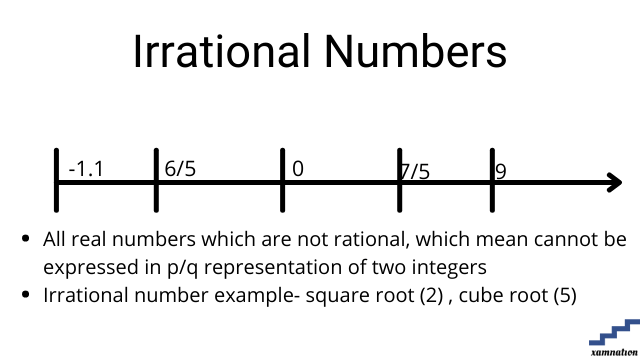# Maths for Grade 8 – Number System | what is Rational number | what is Irrational Integer | what is Counting number

Number system tutorial

Number system is system of representing numbers in mathematics. Students deal with various types of numbers for solving mathematics formulas and calculation, data processing and handling complex topics like algebra and geometry.

In this article, we have shared different types of numbers that are used in mathematics for grade 8 and above. This article can be used by all grades students, as the definition of these different types of numbers is same for all grades.

## What is Rational Number?

### Definition of rational number

• Any number that can be expressed as the quotient or fraction p/q of two integers is called rational number
• It can be both positive and negativeExamples of rational number

• -1.1, 6/5, 9 are example of rational number

Practice question on whole number

Can you find whether below mentioned numbers is rational number or not?

• 10
• 5
• 10/13
• -1.1
• -2/3
• 0
• 305

Send your solution of finding rational numbers to info@xamnation.com , and know detailed answer of this problem.

## What is Irrational number?

### Definition of Irrational number

• ll real numbers which are not rational, which mean cannot be expressed in p/q representation of two integers are called irrational number
• Integers cannot be integer, fraction and decimalExamples of Integer

• Square root(2), cube root(5), Pi are example of irrational number
• 5, 10, 500, 3500 are not irrational number
• -5, -20, -45 are not irrational number
• 6 , 1/3 are not irrational number

Practice question on irrational number

Question: Can you find whether below mentioned number is irrational number or not?

• 10
• Square root(5)
• Pi
• -15
• 10/13
• -1.1

Send your solution of finding irrational number to info@xamnation.com , and know detailed answer of this problem.

## What is Counting number

### Definition of Counting  number

• Any positive integer greater than 0 is counting number .
• Counting numbers are also called Natural numbers
• Counting number example- 1, 5, 50, 300### Examples of counting number

• 1, 5, 10 are example of counting number
• 0, -10, 1/3 are not counting number

Practice question on counting number

Can you find whether below mentioned numbers is Counting number or not?

• 10
• 5
• -15
• 10/13
• -1.1

Send your solution of finding counting number to info@xamnation.com , and know detailed answer of this problem.

## Conclusion

In this article, we have seen how to find whether number is rational number, irrational number or counting number. We also saw detailed examples and practical exercises for number system for grade 8 mathematics.

Are you struck in number system problem or any mathematics topics for grade 8 and above?

You can reach out to us for your math doubts and problem; our math tutors will help you in solving your doubts and take one-one math sessions online. Reach out to us, and our qualified math tutors for grade 8 and above will be glad to help you.